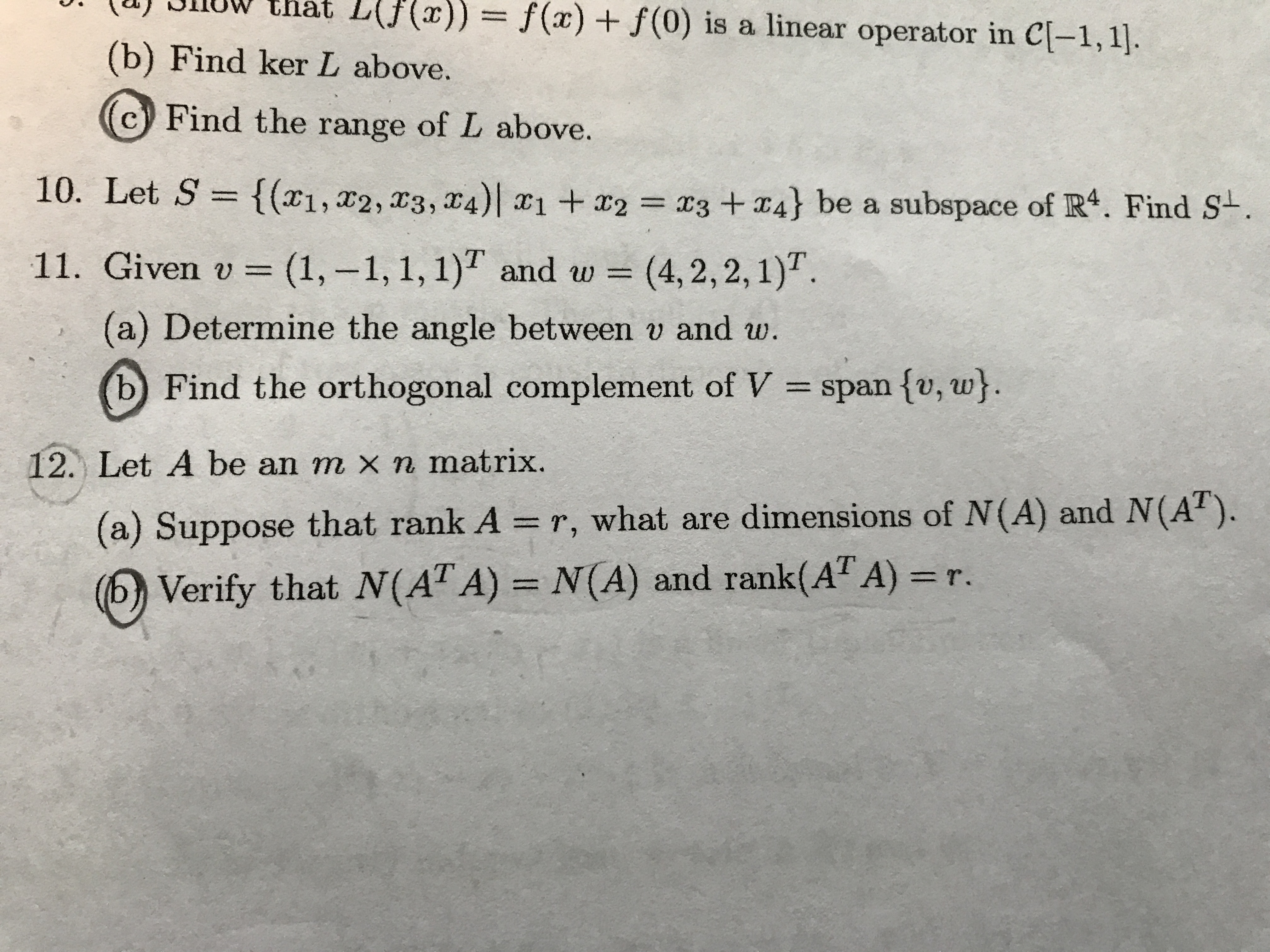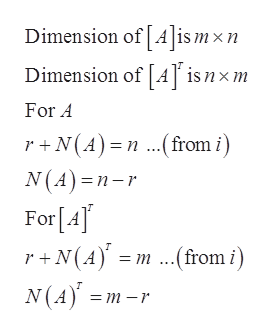# UWthat L(Jf(x))= f(x) f(0) is a linear operator in C[-1,1.(b) Find ker L above.Find the range of L above.10. Let S = {(x1,x2, x3, 4) 1+2x3 +4} be a subspace of R4. Find S-.11. Given v =(1,-1, 1, 1) and w =(4, 2,2,1)(a) Determine the angle between v and w.bFind the orthogonal complement of Vspan fv, w}.12. Let A be an m x n matrix.(a) Suppose that rank A = r, what are dimensions of N(A) and N(A).Verify that N(AT A) = N(A) and rank(AT A) =r.

Question
1 views

#12 a,bhelp_outlineImage TranscriptioncloseUWthat L(Jf(x))= f(x) f(0) is a linear operator in C[-1,1. (b) Find ker L above. Find the range of L above. 10. Let S = {(x1,x2, x3, 4) 1+2 x3 +4} be a subspace of R4. Find S-. 11. Given v =(1,-1, 1, 1) and w = (4, 2,2,1) (a) Determine the angle between v and w. b Find the orthogonal complement of V span fv, w}. 12. Let A be an m x n matrix. (a) Suppose that rank A = r, what are dimensions of N(A) and N(A). Verify that N(AT A) = N(A) and rank(AT A) =r. fullscreen
check_circle

Step 1

Given

Step 2

Concept use

Rank nullity theorem

Step 3

(a) Here...help_outlineImage TranscriptioncloseDimension of [A]is mxn Dimension of [A] is nxm For A r+N(A) n.from i) N(A) n-r For [A] r+N(Am.from i) N(A = m -r fullscreen

### Want to see the full answer?

See Solution

#### Want to see this answer and more?

Solutions are written by subject experts who are available 24/7. Questions are typically answered within 1 hour.*

See Solution
*Response times may vary by subject and question.
Tagged in

### Math